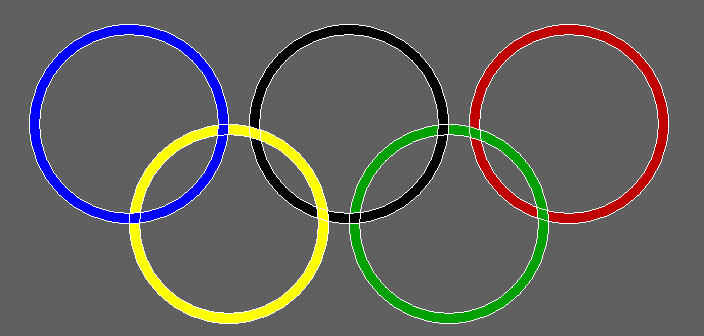Related Articles
C program to draw the Olympics Logo using graphics
• Last Updated : 14 Apr, 2021

In this article, we will discuss how to design the Olympics Logo using Graphics.

#### Approach:

• Draw five circles according to positions in the logo using the function circle().
• To achieve the outline effect, draw 5 smaller circles over it.
• There is a black circle as well in the logo and to prevent it from blending in, change the background color.
• Color all the circles and the background to their respective colors using functions setfillstyle() and floodfill().

Below is the implementation of the above approach:

## C

 `// C program for the above approach`` ` `#include ``#include ``#include `` ` `// Driver Code``void` `main()``{``    ``int` `gd = DETECT, gm;`` ` `    ``// Initialize of gdriver``    ``initgraph(&gd, &gm, ``"C:\\turboc3\\bgi"``);`` ` `    ``// Create Background color as Grey``    ``setfillstyle(SOLID_FILL, DARKGRAY);``    ``floodfill(50, 50, 15);`` ` `    ``// Create two circles in each``    ``// another & color Blue``    ``setfillstyle(SOLID_FILL, BLUE);``    ``circle(300, 300, 100);``    ``circle(300, 300, 90);``    ``floodfill(202, 300, 15);`` ` `    ``// Create two circles in each``    ``// another & color Yellow``    ``setfillstyle(SOLID_FILL, YELLOW);``    ``circle(400, 400, 100);``    ``circle(400, 400, 90);``    ``floodfill(322, 350, 15);``    ``floodfill(302, 400, 15);`` ` `    ``// Create two circles in each``    ``// another & color Black``    ``setfillstyle(SOLID_FILL, BLACK);``    ``circle(520, 300, 100);``    ``circle(520, 300, 90);``    ``floodfill(442, 350, 15);``    ``floodfill(422, 300, 15);`` ` `    ``// Create two circles in each``    ``// another & color Green``    ``setfillstyle(SOLID_FILL, GREEN);``    ``circle(620, 400, 100);``    ``circle(620, 400, 90);``    ``floodfill(522, 400, 15);``    ``floodfill(542, 350, 15);`` ` `    ``// Create two circles in each``    ``// another & color Red``    ``setfillstyle(SOLID_FILL, RED);``    ``circle(740, 300, 100);``    ``circle(740, 300, 90);``    ``floodfill(642, 300, 15);``    ``floodfill(662, 350, 15);`` ` `    ``// Hold the screen for a while``    ``getch();`` ` `    ``// Close the initialized gdriver``    ``closegraph();``}`

Output:Want to learn from the best curated videos and practice problems, check out the C Foundation Course for Basic to Advanced C.

My Personal Notes arrow_drop_up